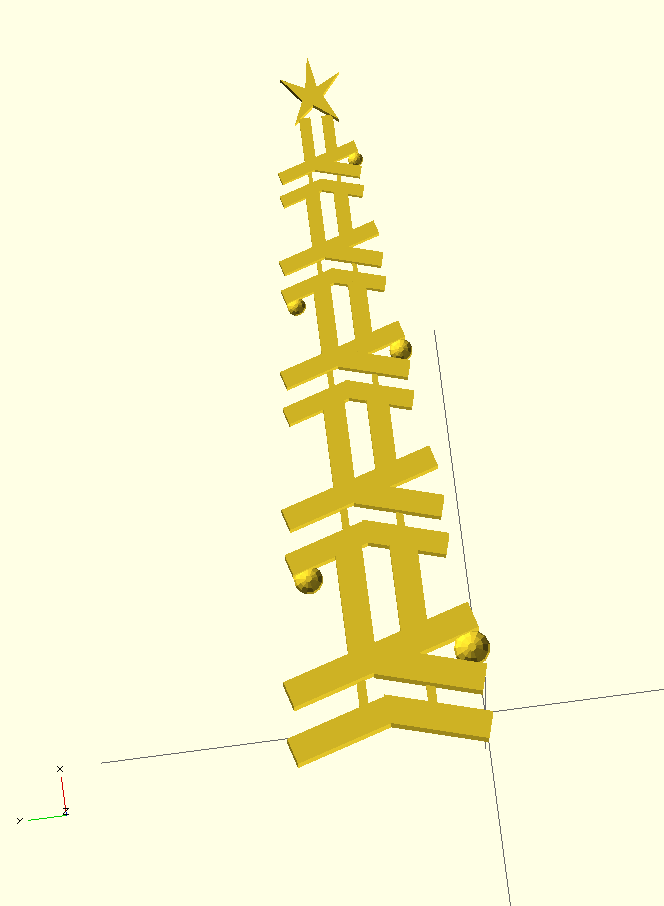Posted on December 18, 2015 by Noon van der Silk

At the last Melbourne Haskell Meetup we got into the spirit by making Christmas trees in Haskell.

However, I recently have access to a 3D printer, and I’ve long wanted an excuse to try and use ImplicitCAD, so I set about trying to make a 3D version of Lyndon’s logo.

So of course, I love stack I created a new simple project with stack new and got started.

It turns out that ImplicitCAD has a pretty nice and reasonably intuitive interface (similar to the code that one would write into OpenSCAD).

I built the 3D version of the logo by moving around rectangles, and by extruding a hand-drawn shape.

The cool thing about generating this image in fully-feature programming language is that I can build a tree of any size I like!

Here’s the (pretty verbose) code that gets me a tree of arbitrary depth:

ntree :: Integer -> SymbolicObj3
ntree n = finalObj
where
dec     = 0.8
ratios  = 0 : [dec^j | j <- [0..(n-2)]]
-- build up logo structure
((lx, ly, lz), objs) = foldl f ((0, 0, 0), []) (zip [0..(n-1)] ratios)
-- position of logos
(x,y,z) = (40, 4, 0)
f :: ((ℝ, ℝ, ℝ), [SymbolicObj3]) -> (Integer, Float) -> ((ℝ, ℝ, ℝ), [SymbolicObj3])
f ((x', y', z'), xs) (j, r) =
let newPos = (x' + r*x, y' + r*y, z' + r*z)
s      = dec ^ j
loc    = if (even j) then R else L
obj3   = translate newPos $scale (s, s, s) (logoBauble loc) in (newPos, obj3 : xs) -- star (a,b,c) = (40.5, 24.5, 0) starScale = dec ** (fromIntegral (n-3)) posScale = dec ** (fromIntegral n) starObj = translate (lx + (posScale * a), ly + (posScale * b), lz + (posScale * c))$ scale (starScale, starScale, starScale) star
finalObj = union (starObj : objs)

Running this with n = 5 gives result in the following render in OpenSCAD: